# RD Sharma Solutions for Class 8 Maths Chapter 6 - Algebraic Expressions and Identities Exercise 6.6

The solutions for RD Sharma Class 8 Maths Exercise 6.6 of Chapter 6 Algebraic Expressions and Identities are provided here in pdf format. BYJU’S experts have formulated these solutions which help students improve their conceptual knowledge on the concepts to obtain good marks in their exams. To excel in their board exams, we suggest students practice the RD Sharma Solutions thoroughly on a regular basis. The pdfs can be downloaded easily from the links available below. In Exercise 6.6 of Chapter 6 algebraic expressions and identities, we shall study problems based on the identities (identity is an equality which is true for all values of the variable).

## Download the pdf of RD Sharma Solutions For Class 8 Maths Exercise 6.6 Chapter 6 Algebraic Expressions and Identities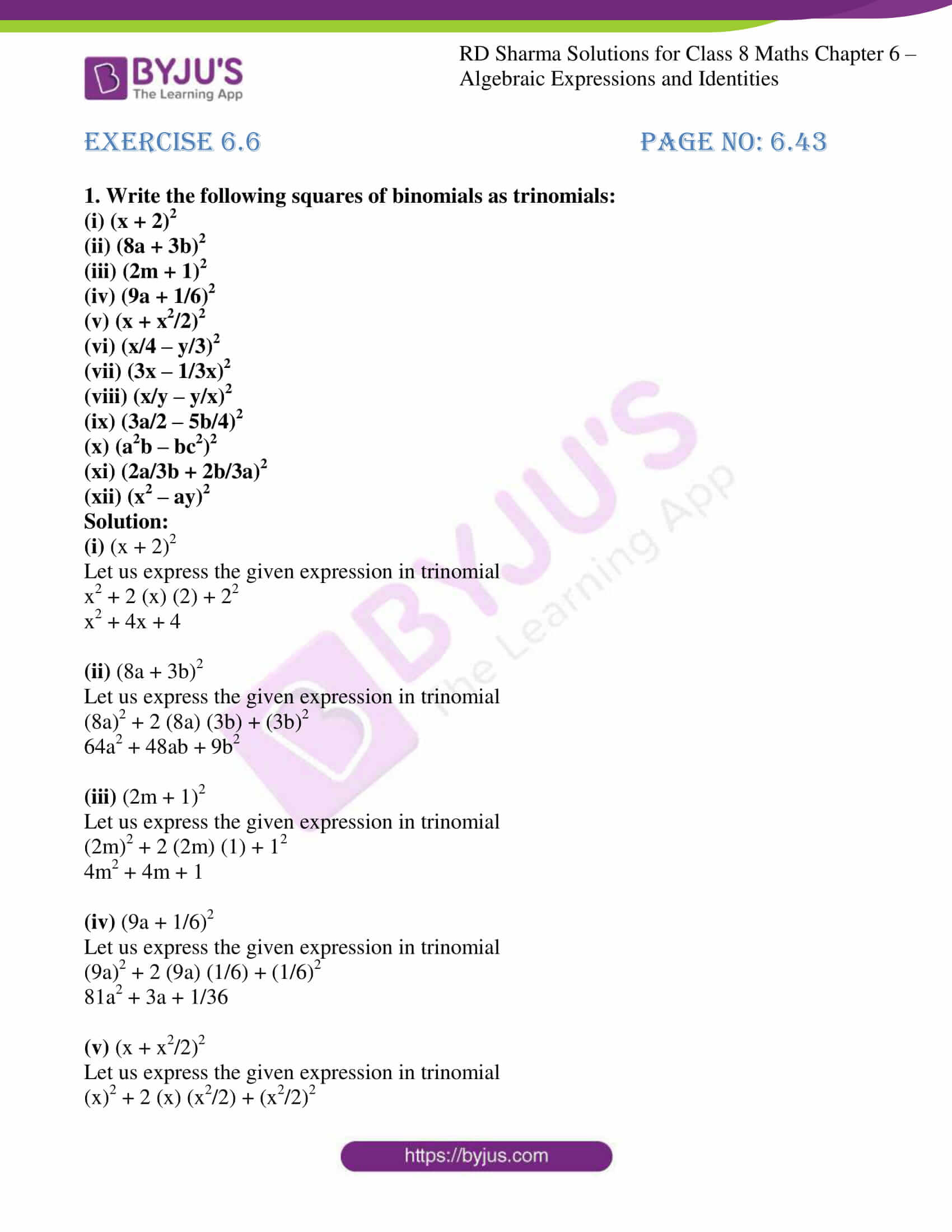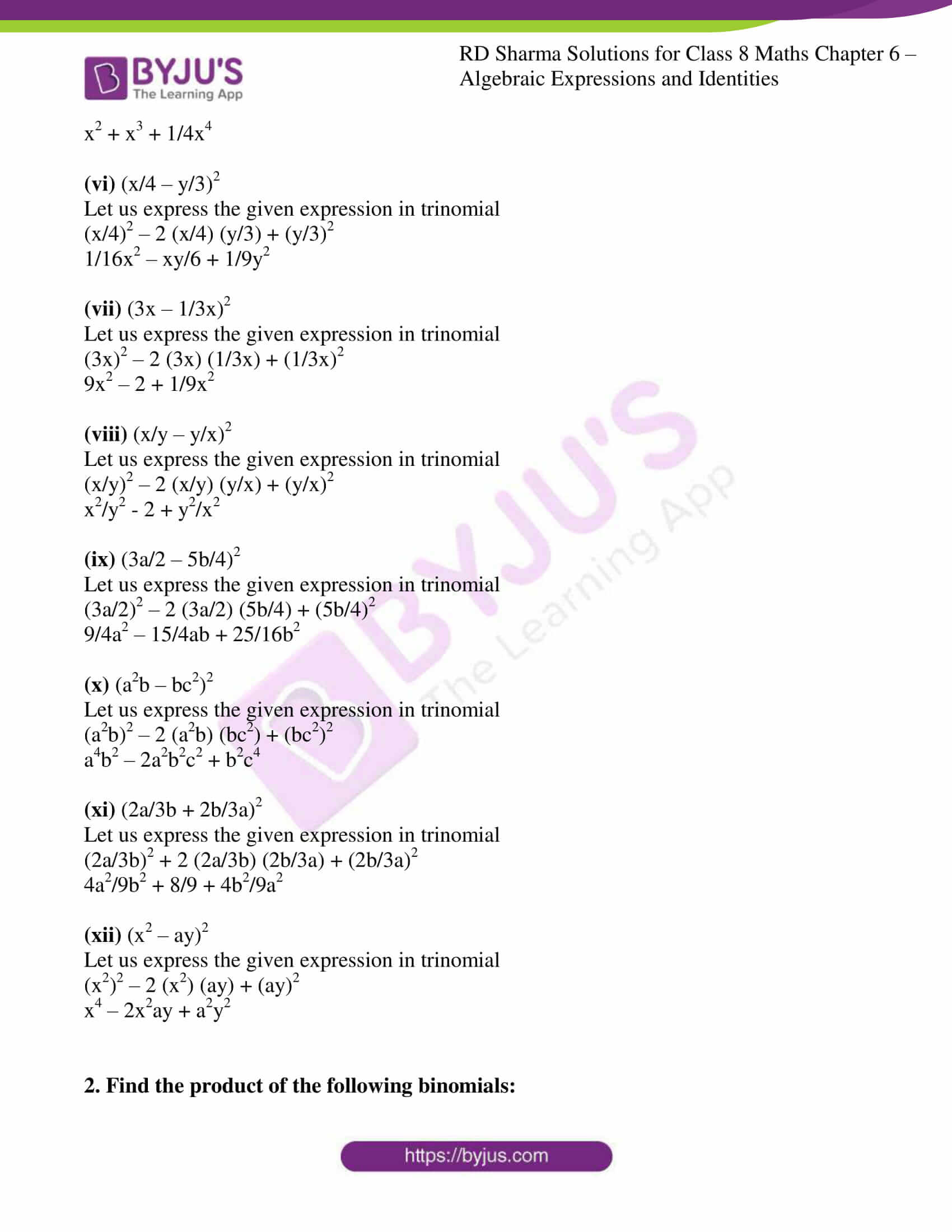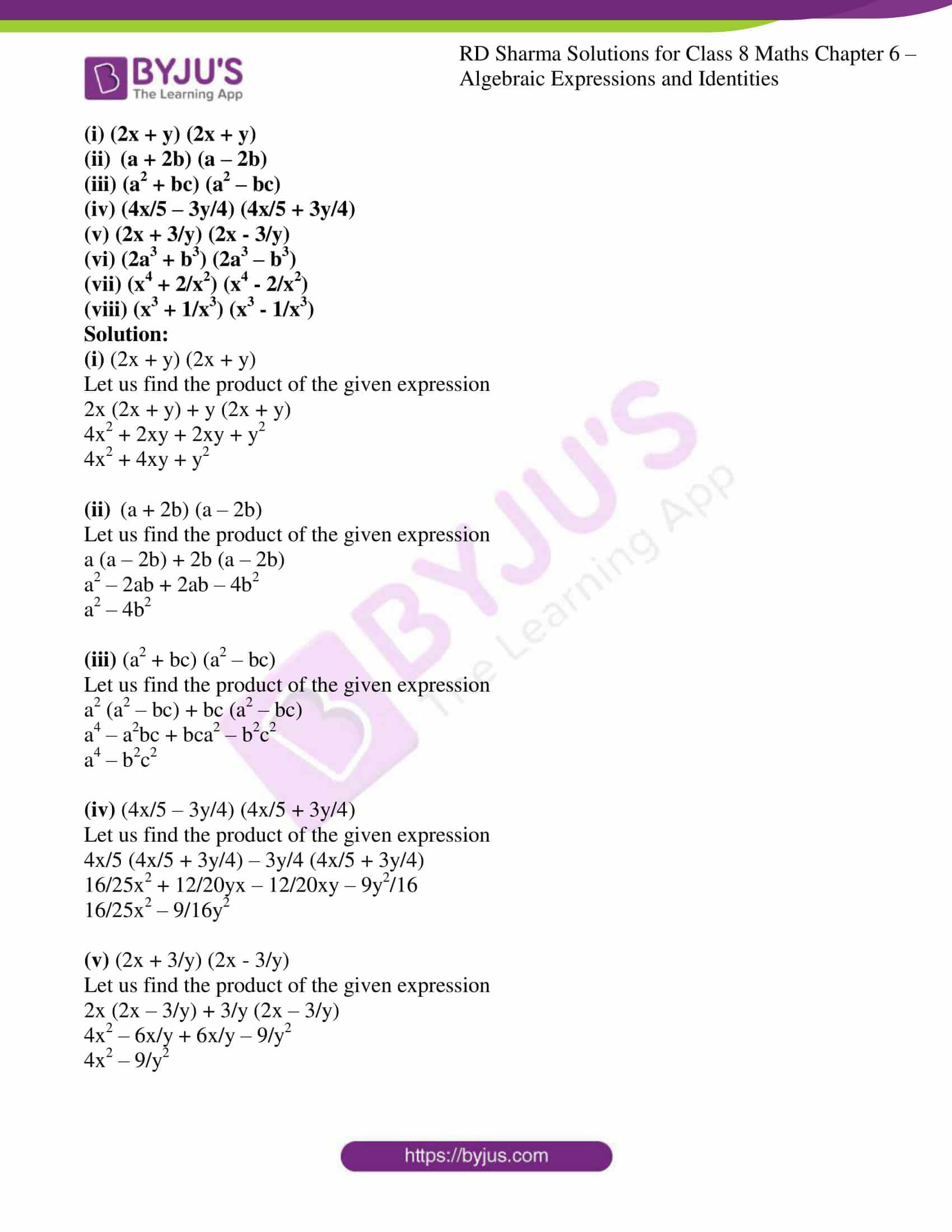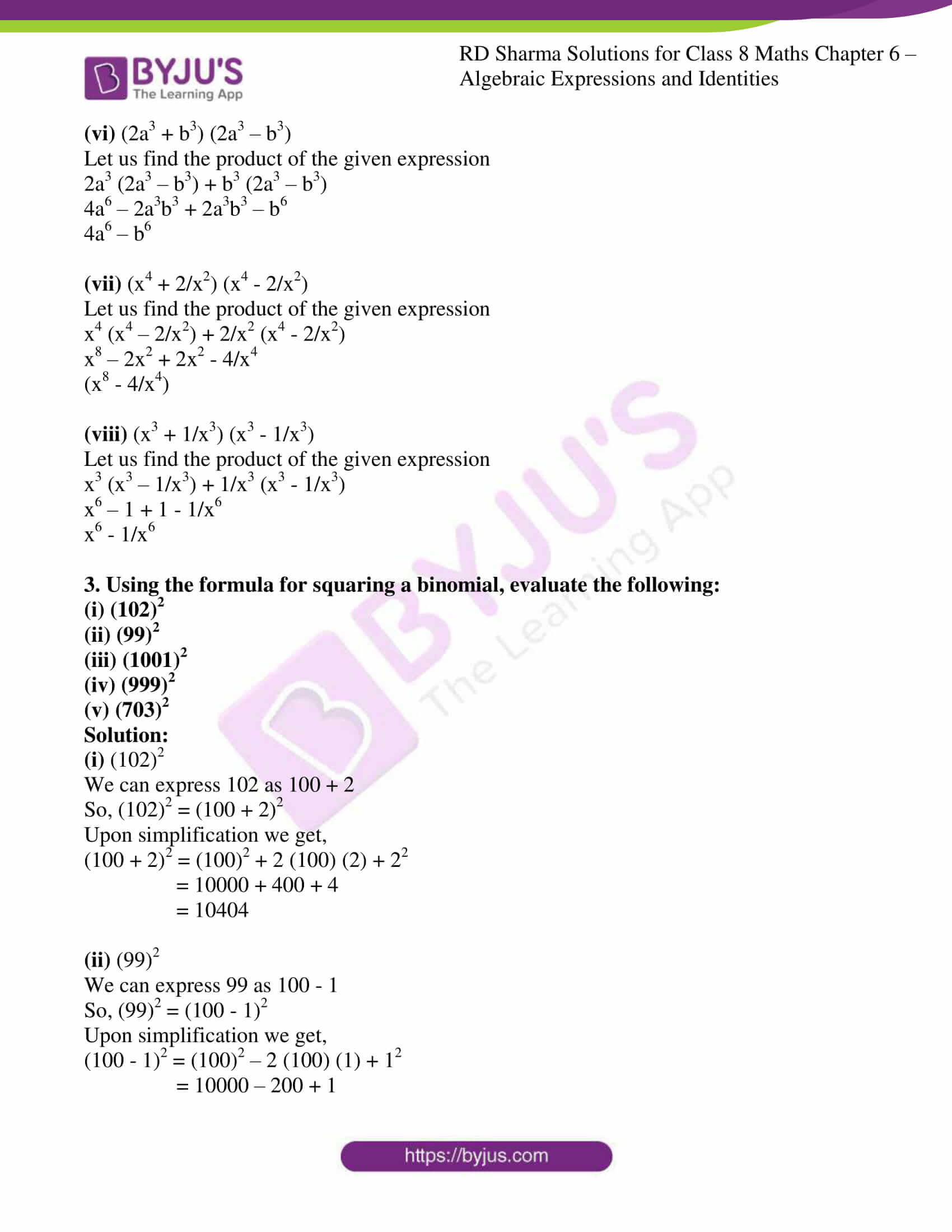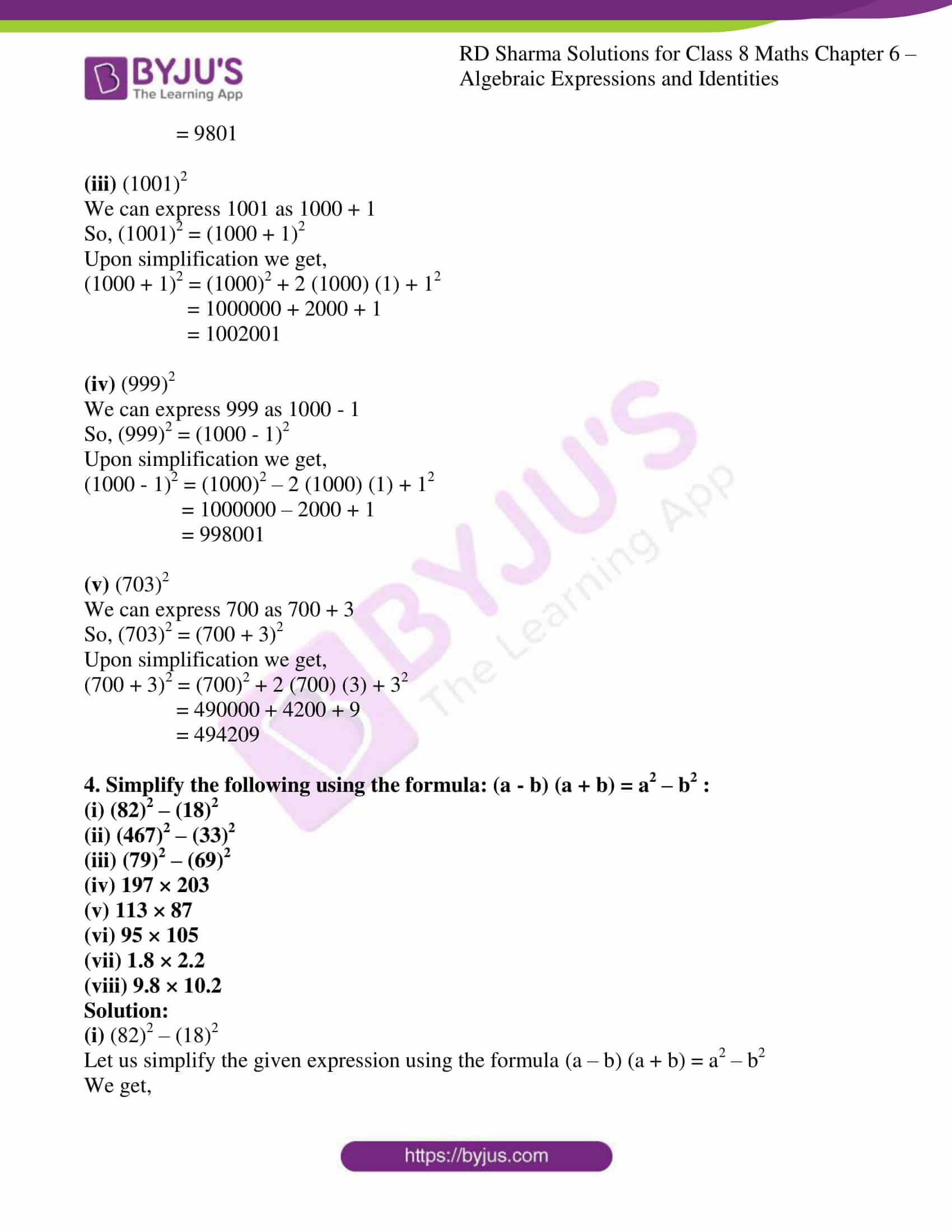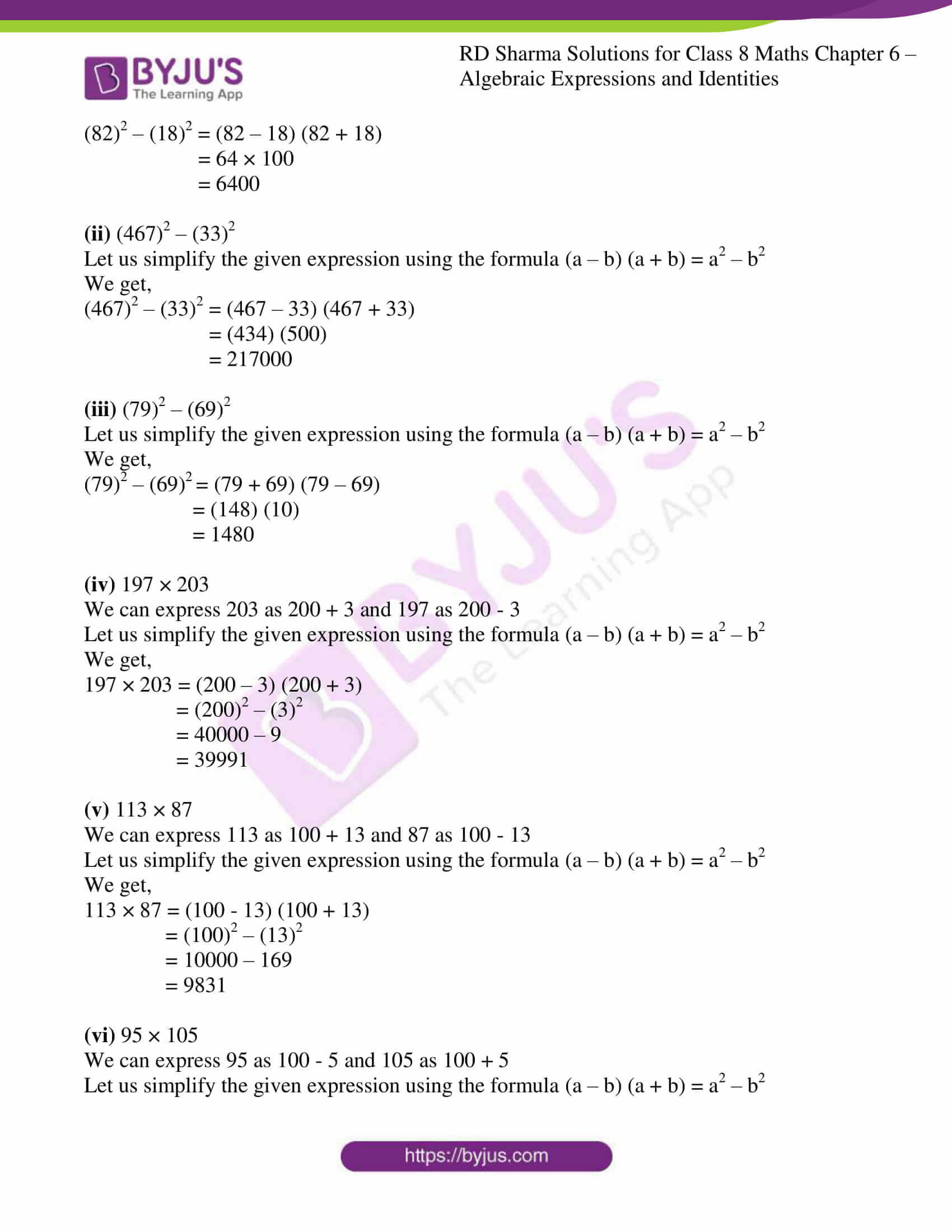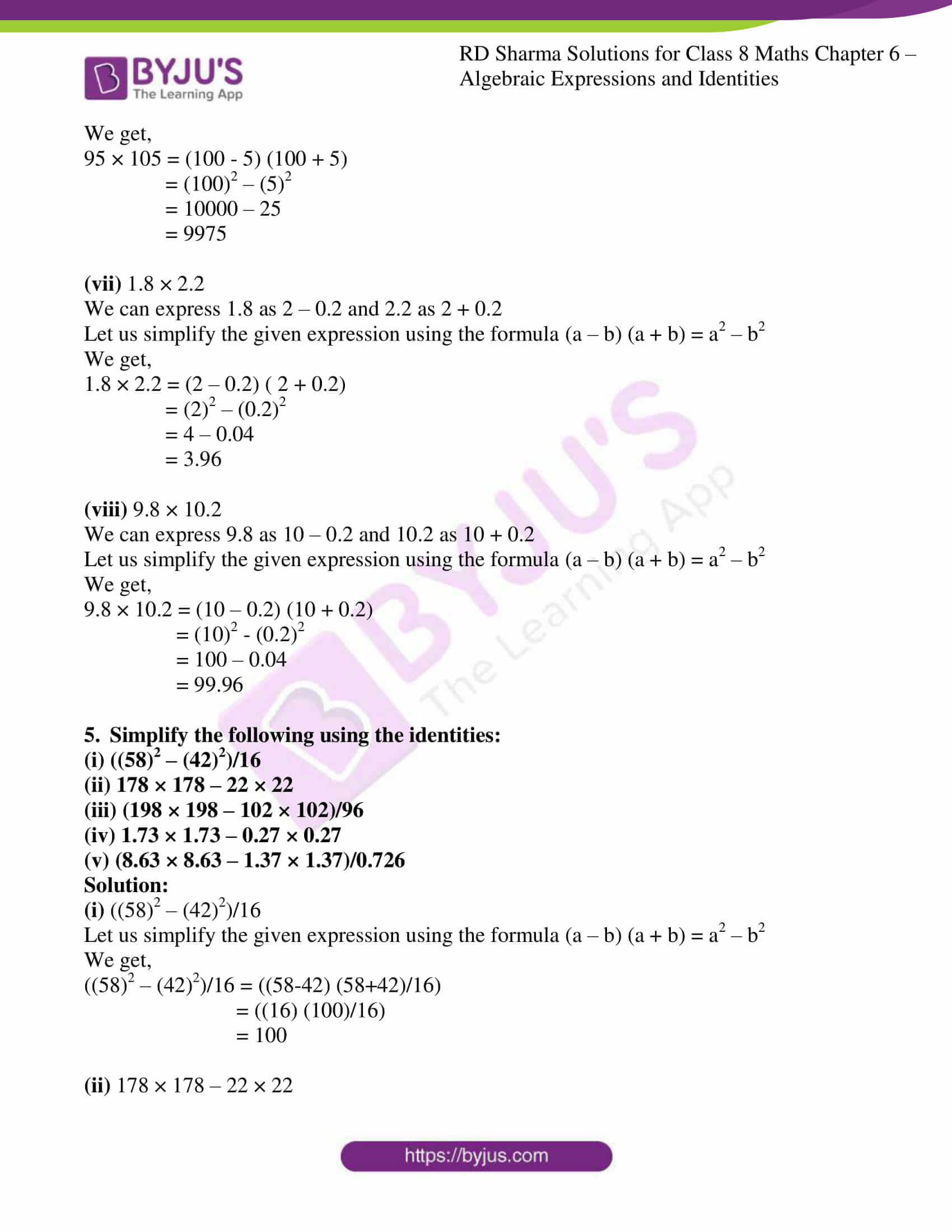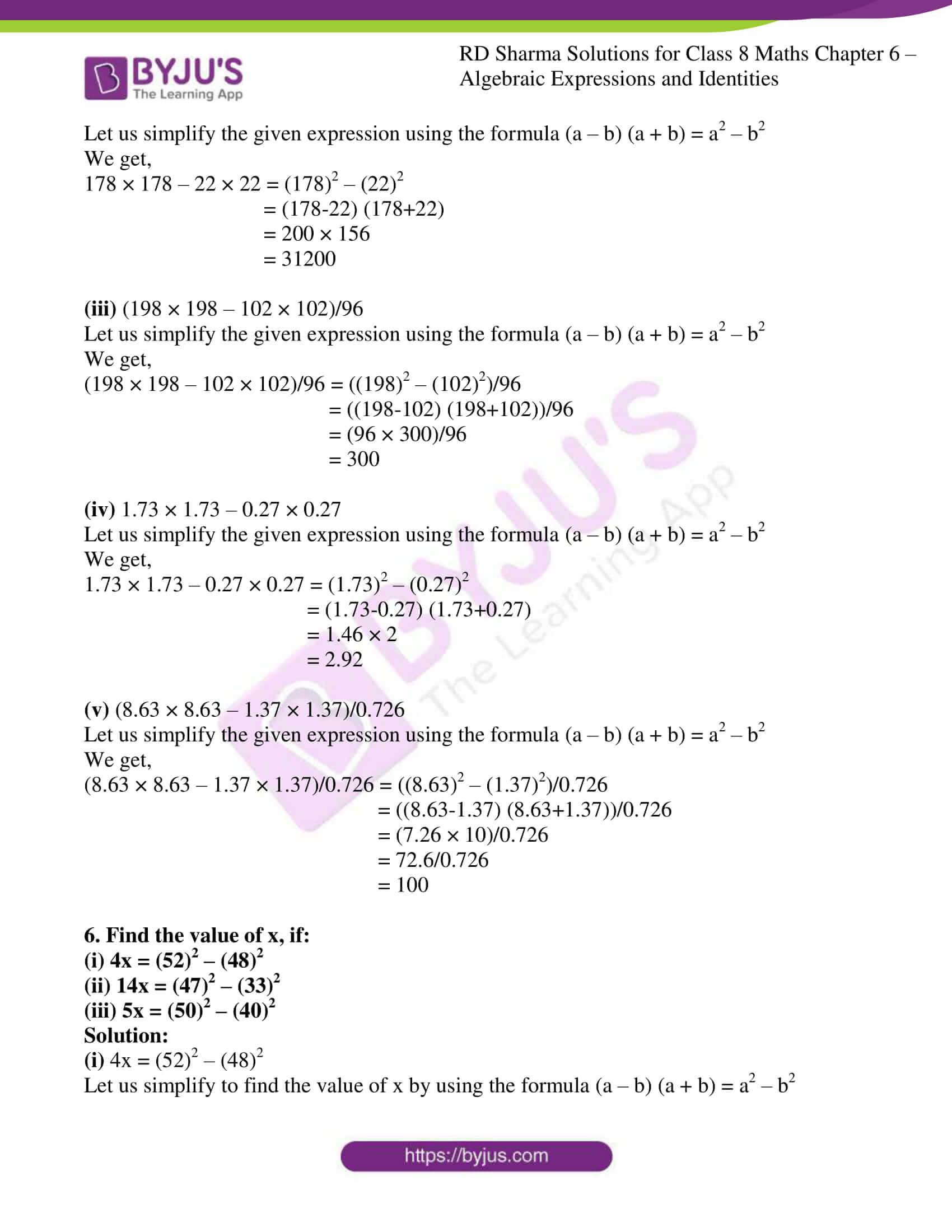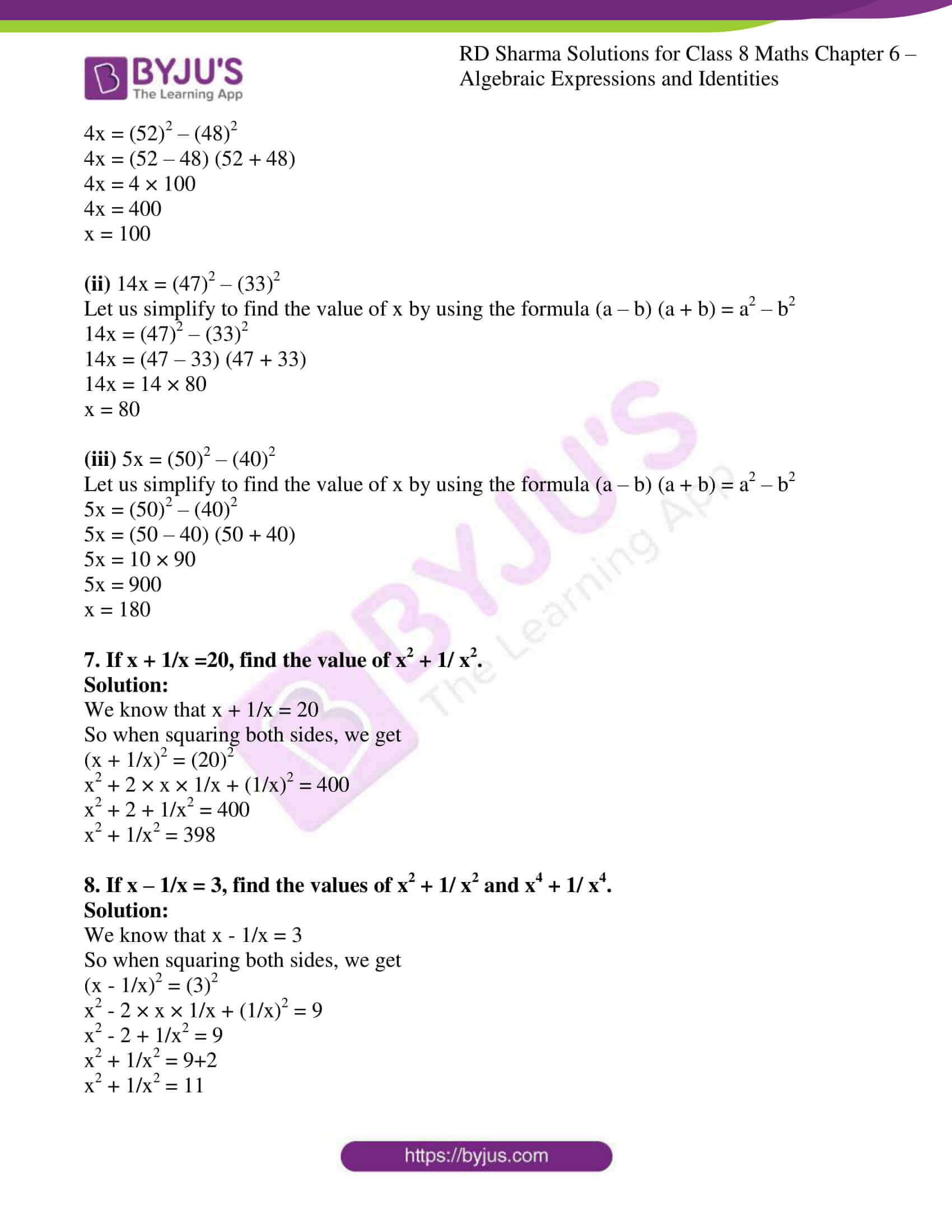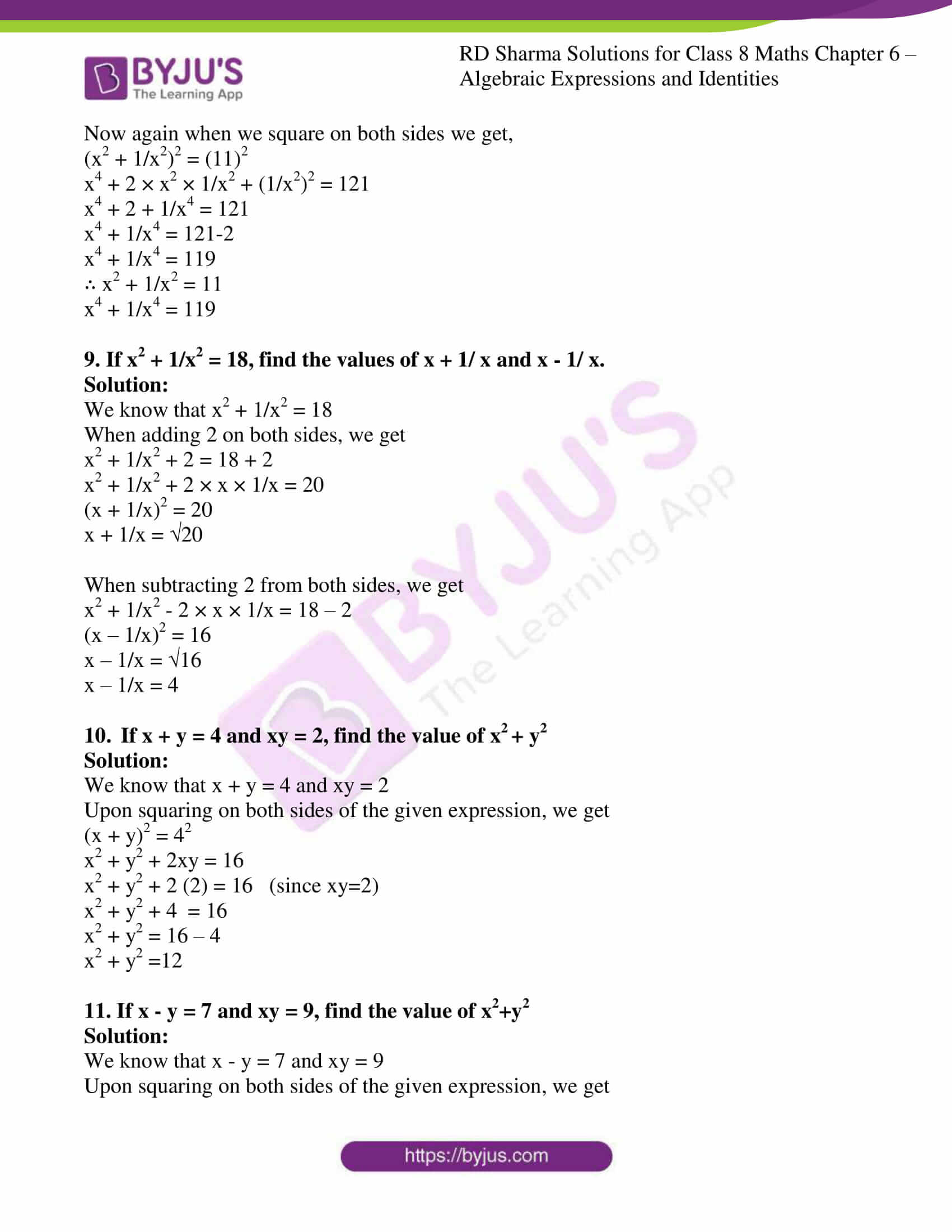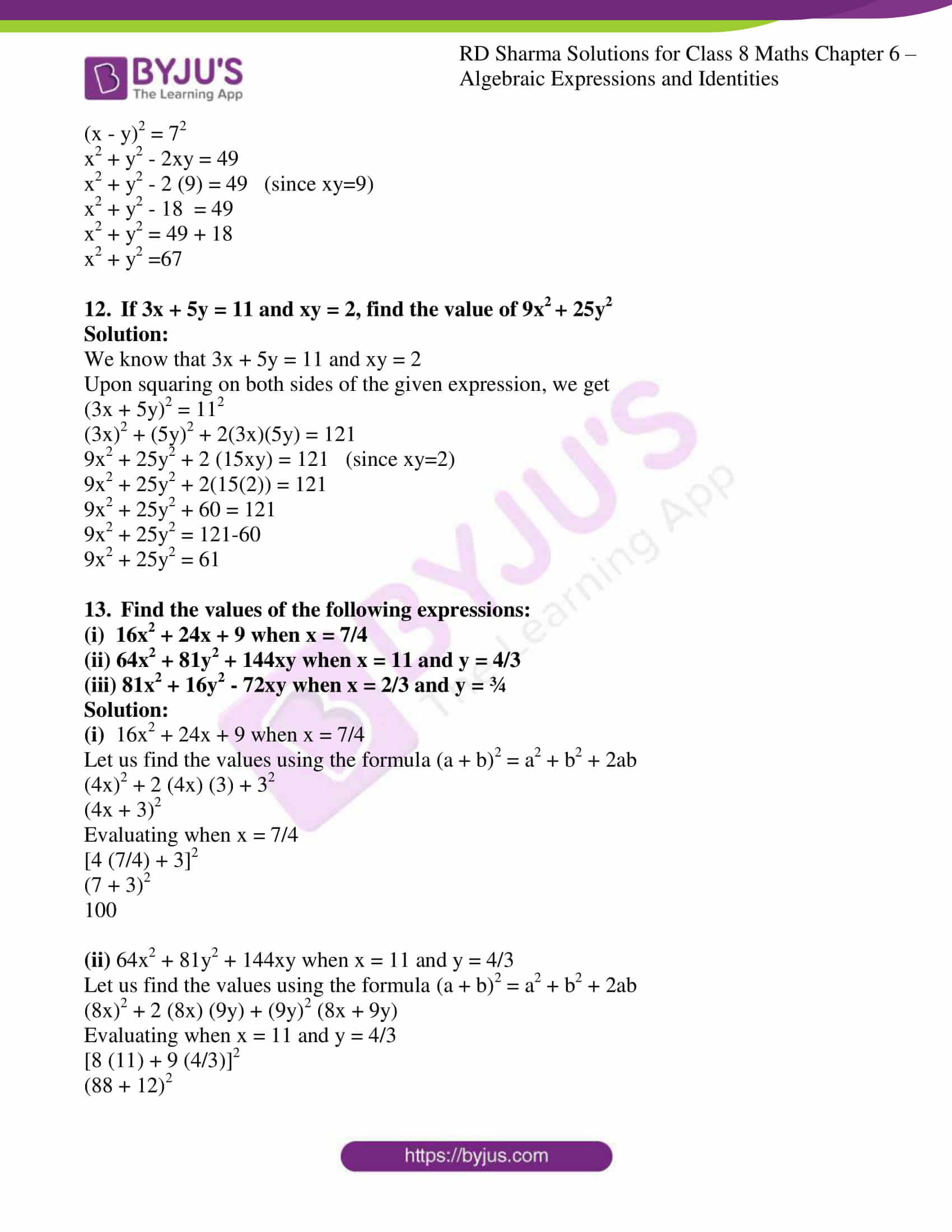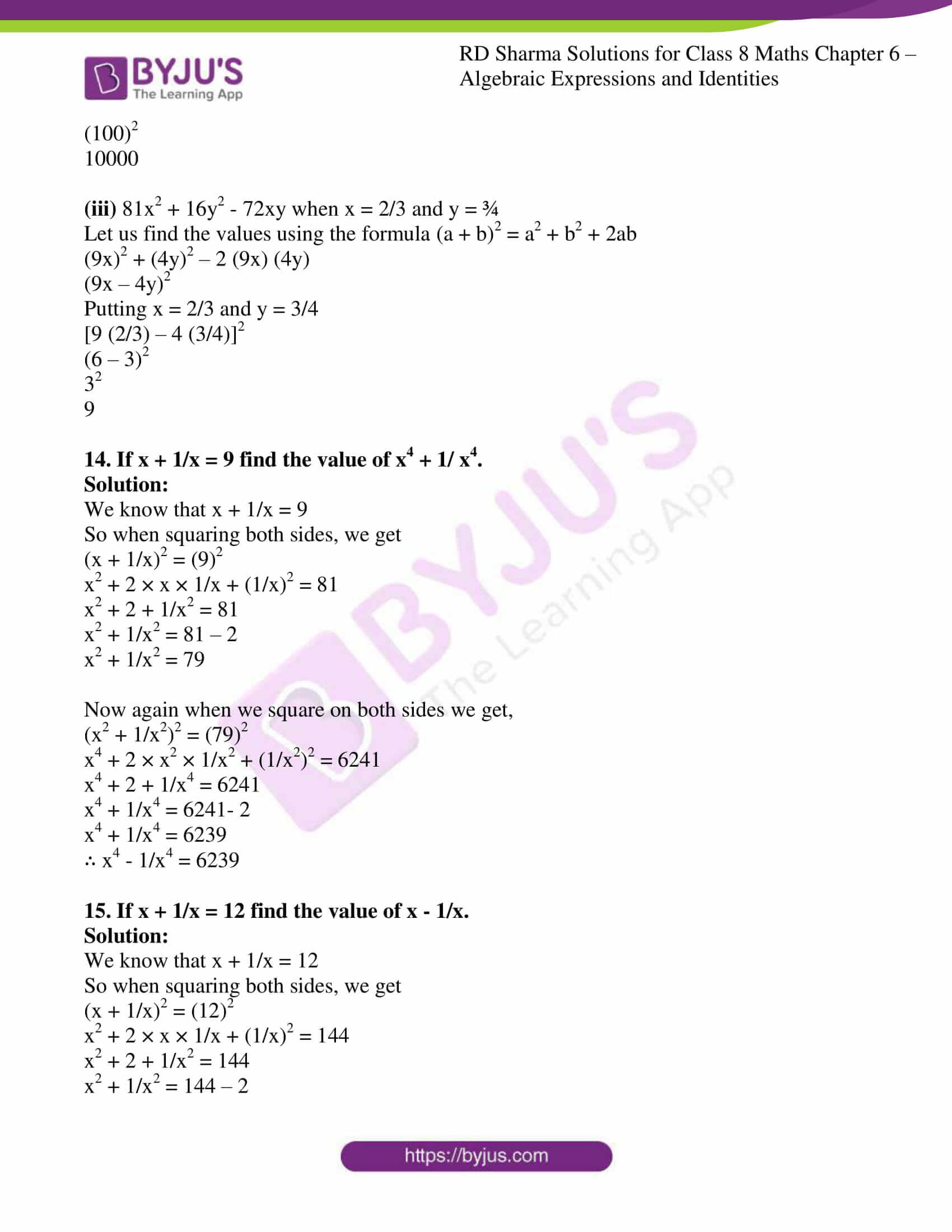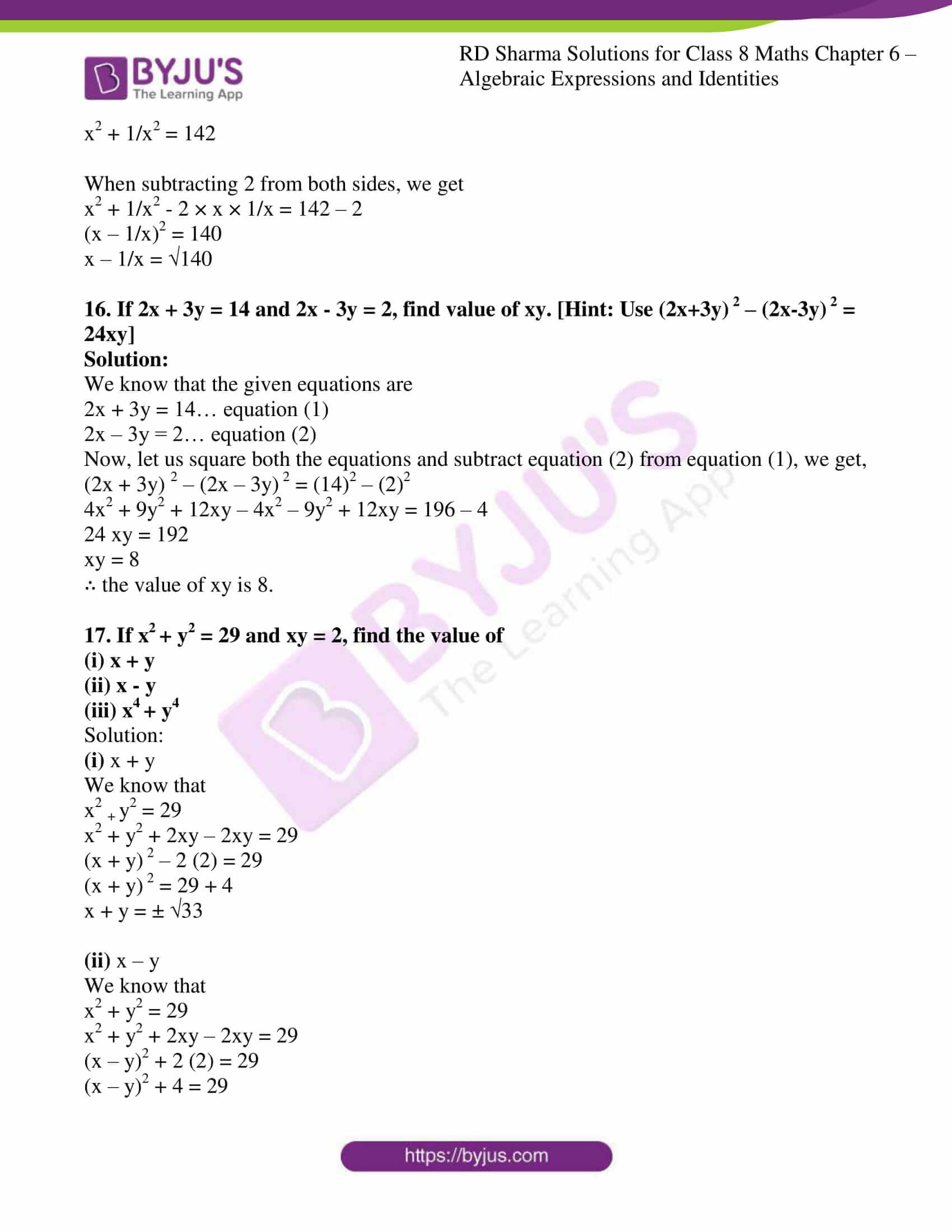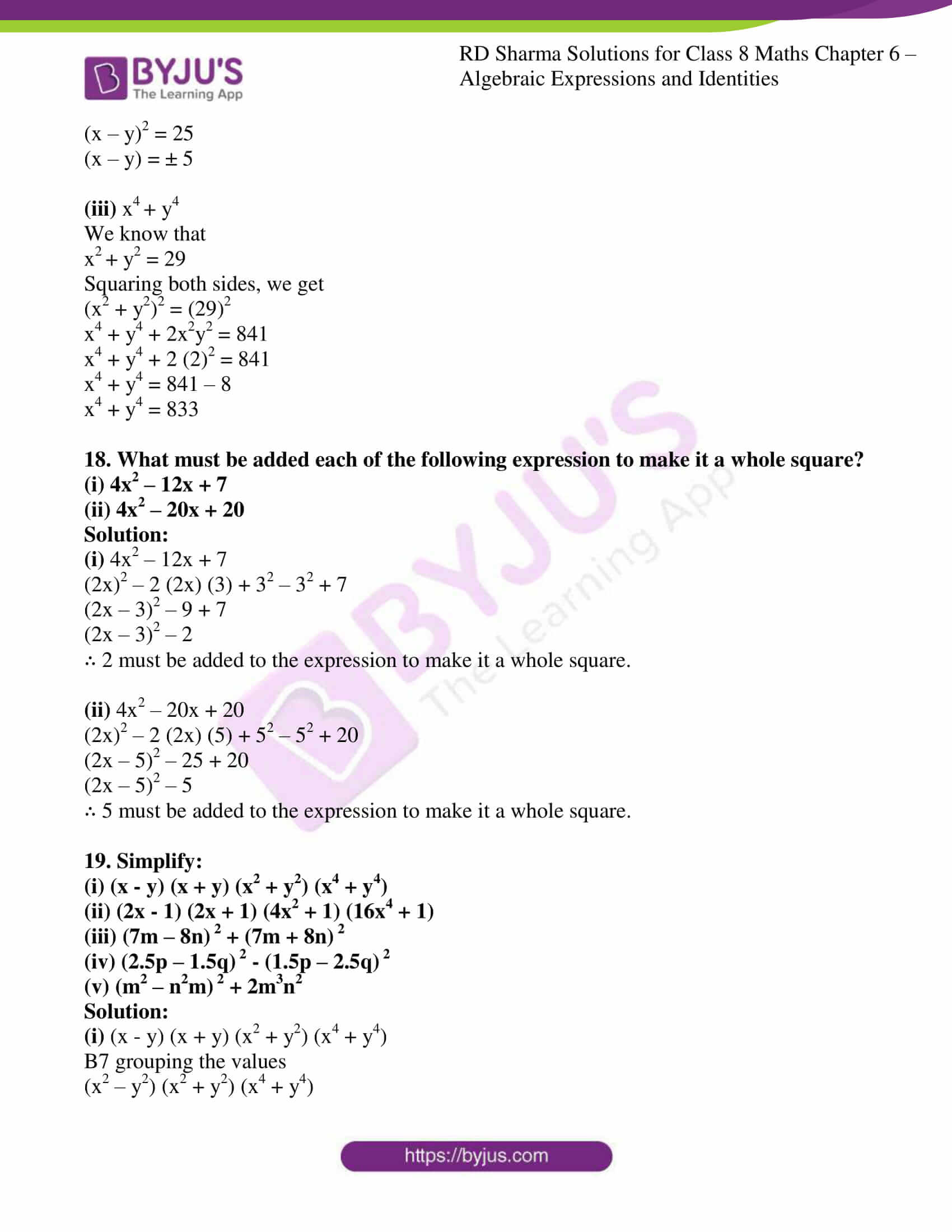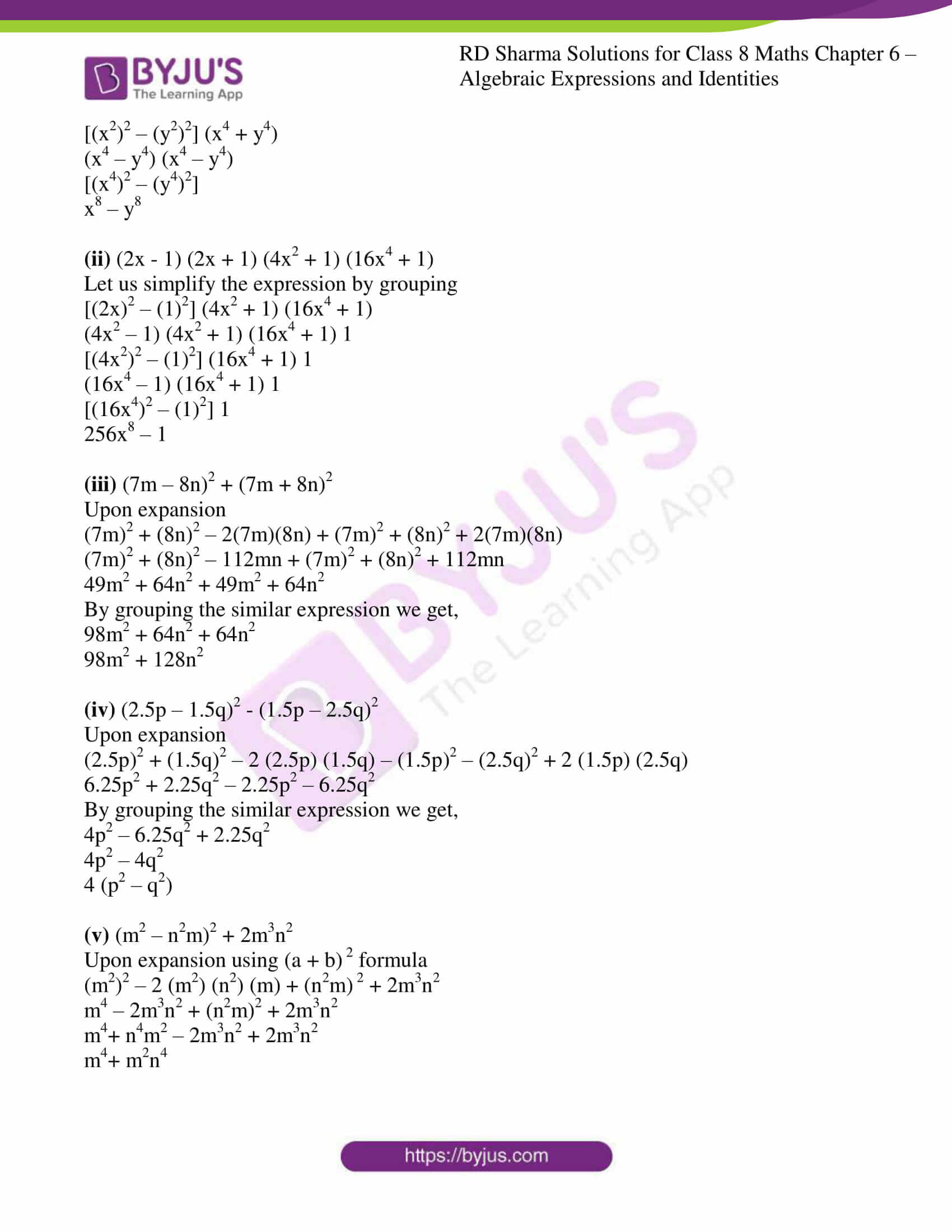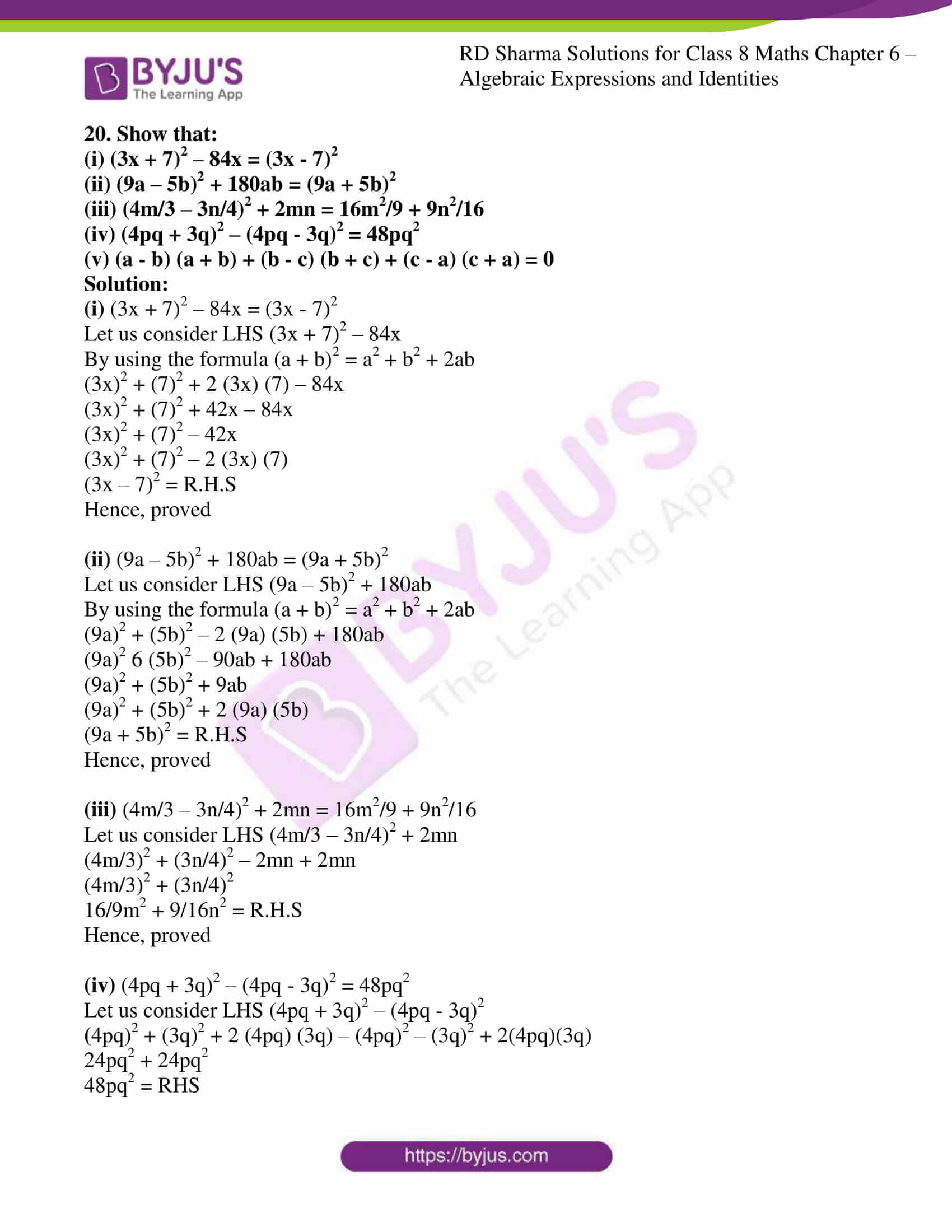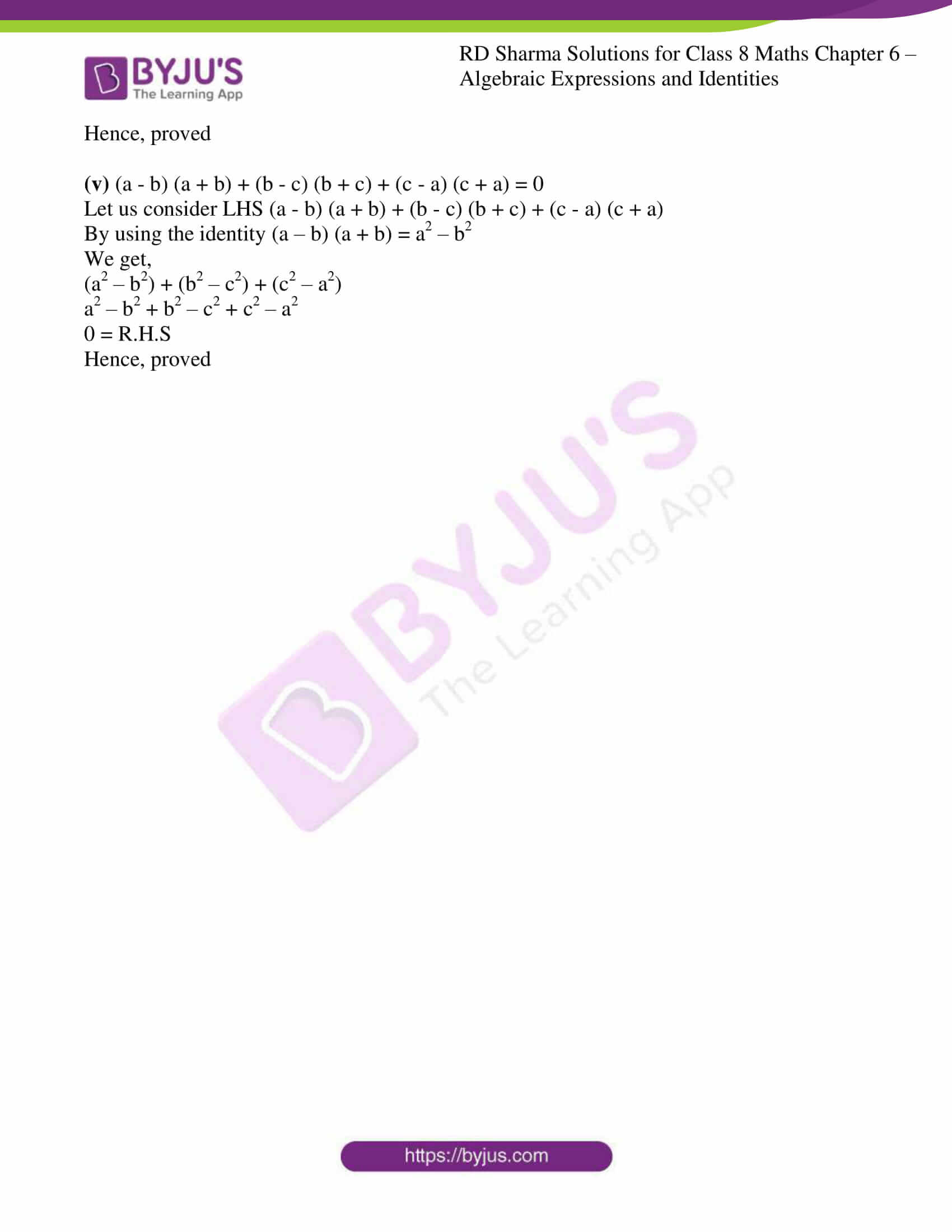### Access Answers to RD Sharma Solutions for Class 8 Maths Exercise 6.6 Chapter 6 Algebraic Expressions and Identities

#### EXERCISE 6.6 PAGE NO: 6.43

1. Write the following squares of binomials as trinomials:

(i) (x + 2)2

(ii) (8a + 3b)2

(iii) (2m + 1)2

(iv) (9a + 1/6)2

(v) (x + x2/2)2

(vi) (x/4 – y/3)2

(vii) (3x – 1/3x)2

(viii) (x/y – y/x)2

(ix) (3a/2 – 5b/4)2

(x) (a2b – bc2)2

(xi) (2a/3b + 2b/3a)2

(xii) (x2 – ay)2

Solution:

(i) (x + 2)2

Let us express the given expression in trinomial

x2 + 2 (x) (2) + 22

x2 + 4x + 4

(ii) (8a + 3b)2

Let us express the given expression in trinomial

(8a)2 + 2 (8a) (3b) + (3b)2

64a2 + 48ab + 9b2

(iii) (2m + 1)2

Let us express the given expression in trinomial

(2m)2 + 2 (2m) (1) + 12

4m2 + 4m + 1

(iv) (9a + 1/6)2

Let us express the given expression in trinomial

(9a)2 + 2 (9a) (1/6) + (1/6)2

81a2 + 3a + 1/36

(v) (x + x2/2)2

Let us express the given expression in trinomial

(x)2 + 2 (x) (x2/2) + (x2/2)2

x2 + x3 + 1/4x4

(vi) (x/4 – y/3)2

Let us express the given expression in trinomial

(x/4)2 – 2 (x/4) (y/3) + (y/3)2

1/16x2 – xy/6 + 1/9y2

(vii) (3x – 1/3x)2

Let us express the given expression in trinomial

(3x)2 – 2 (3x) (1/3x) + (1/3x)2

9x2 – 2 + 1/9x2

(viii) (x/y – y/x)2

Let us express the given expression in trinomial

(x/y)2 – 2 (x/y) (y/x) + (y/x)2

x2/y2 – 2 + y2/x2

(ix) (3a/2 – 5b/4)2

Let us express the given expression in trinomial

(3a/2)2 – 2 (3a/2) (5b/4) + (5b/4)2

9/4a2 – 15/4ab + 25/16b2

(x) (a2b – bc2)2

Let us express the given expression in trinomial

(a2b)2 – 2 (a2b) (bc2) + (bc2)2

a4b2 – 2a2b2c2 + b2c4

(xi) (2a/3b + 2b/3a)2

Let us express the given expression in trinomial

(2a/3b)2 + 2 (2a/3b) (2b/3a) + (2b/3a)2

4a2/9b2 + 8/9 + 4b2/9a2

(xii) (x2 – ay)2

Let us express the given expression in trinomial

(x2)2 – 2 (x2) (ay) + (ay)2

x4 – 2x2ay + a2y2

2. Find the product of the following binomials:

(i) (2x + y) (2x + y)

(ii) (a + 2b) (a – 2b)

(iii) (a2 + bc) (a2 – bc)

(iv) (4x/5 – 3y/4) (4x/5 + 3y/4)

(v) (2x + 3/y) (2x – 3/y)

(vi) (2a3 + b3) (2a3 – b3)

(vii) (x4 + 2/x2) (x4 – 2/x2)

(viii) (x3 + 1/x3) (x3 – 1/x3)

Solution:

(i) (2x + y) (2x + y)

Let us find the product of the given expression

2x (2x + y) + y (2x + y)

4x2 + 2xy + 2xy + y2

4x2 + 4xy + y2

(ii) (a + 2b) (a – 2b)

Let us find the product of the given expression

a (a – 2b) + 2b (a – 2b)

a2 – 2ab + 2ab – 4b2

a2 – 4b2

(iii) (a2 + bc) (a2 – bc)

Let us find the product of the given expression

a2 (a2 – bc) + bc (a2 – bc)

a4 – a2bc + bca2 – b2c2

a4 – b2c2

(iv) (4x/5 – 3y/4) (4x/5 + 3y/4)

Let us find the product of the given expression

4x/5 (4x/5 + 3y/4) – 3y/4 (4x/5 + 3y/4)

16/25x2 + 12/20yx – 12/20xy – 9y2/16

16/25x2 – 9/16y2

(v) (2x + 3/y) (2x – 3/y)

Let us find the product of the given expression

2x (2x – 3/y) + 3/y (2x – 3/y)

4x2 – 6x/y + 6x/y – 9/y2

4x2 – 9/y2

(vi) (2a3 + b3) (2a3 – b3)

Let us find the product of the given expression

2a3 (2a3 – b3) + b3 (2a3 – b3)

4a6 – 2a3b3 + 2a3b3 – b6

4a6 – b6

(vii) (x4 + 2/x2) (x4 – 2/x2)

Let us find the product of the given expression

x4 (x4 – 2/x2) + 2/x2 (x4 – 2/x2)

x8 – 2x2 + 2x2 – 4/x4

(x8 – 4/x4)

(viii) (x3 + 1/x3) (x3 – 1/x3)

Let us find the product of the given expression

x3 (x3 – 1/x3) + 1/x3 (x3 – 1/x3)

x6 – 1 + 1 – 1/x6

x6 – 1/x6

3. Using the formula for squaring a binomial, evaluate the following:

(i) (102)2

(ii) (99)2

(iii) (1001)2

(iv) (999)2

(v) (703)2

Solution:

(i) (102)2

We can express 102 as 100 + 2

So, (102)2 = (100 + 2)2

Upon simplification we get,

(100 + 2)2 = (100)2 + 2 (100) (2) + 22

= 10000 + 400 + 4

= 10404

(ii) (99)2

We can express 99 as 100 – 1

So, (99)2 = (100 – 1)2

Upon simplification we get,

(100 – 1)2 = (100)2 – 2 (100) (1) + 12

= 10000 – 200 + 1

= 9801

(iii) (1001)2

We can express 1001 as 1000 + 1

So, (1001)2 = (1000 + 1)2

Upon simplification we get,

(1000 + 1)2 = (1000)2 + 2 (1000) (1) + 12

= 1000000 + 2000 + 1

= 1002001

(iv) (999)2

We can express 999 as 1000 – 1

So, (999)2 = (1000 – 1)2

Upon simplification we get,

(1000 – 1)2 = (1000)2 – 2 (1000) (1) + 12

= 1000000 – 2000 + 1

= 998001

(v) (703)2

We can express 700 as 700 + 3

So, (703)2 = (700 + 3)2

Upon simplification we get,

(700 + 3)2 = (700)2 + 2 (700) (3) + 32

= 490000 + 4200 + 9

= 494209

4. Simplify the following using the formula: (a – b) (a + b) = a2 – b2 :

(i) (82)2 – (18)2

(ii) (467)2 – (33)2

(iii) (79)2 – (69)2

(iv) 197 × 203

(v) 113 × 87

(vi) 95 × 105

(vii) 1.8 × 2.2

(viii) 9.8 × 10.2

Solution:

(i) (82)2 – (18)2

Let us simplify the given expression using the formula (a – b) (a + b) = a2 – b2

We get,

(82)2 – (18)2 = (82 – 18) (82 + 18)

= 64 × 100

= 6400

(ii) (467)2 – (33)2

Let us simplify the given expression using the formula (a – b) (a + b) = a2 – b2

We get,

(467)2 – (33)2 = (467 – 33) (467 + 33)

= (434) (500)

= 217000

(iii) (79)2 – (69)2

Let us simplify the given expression using the formula (a – b) (a + b) = a2 – b2

We get,

(79)2 – (69)2 = (79 + 69) (79 – 69)

= (148) (10)

= 1480

(iv) 197 × 203

We can express 203 as 200 + 3 and 197 as 200 – 3

Let us simplify the given expression using the formula (a – b) (a + b) = a2 – b2

We get,

197 × 203 = (200 – 3) (200 + 3)

= (200)2 – (3)2

= 40000 – 9

= 39991

(v) 113 × 87

We can express 113 as 100 + 13 and 87 as 100 – 13

Let us simplify the given expression using the formula (a – b) (a + b) = a2 – b2

We get,

113 × 87 = (100 – 13) (100 + 13)

= (100)2 – (13)2

= 10000 – 169

= 9831

(vi) 95 × 105

We can express 95 as 100 – 5 and 105 as 100 + 5

Let us simplify the given expression using the formula (a – b) (a + b) = a2 – b2

We get,

95 × 105 = (100 – 5) (100 + 5)

= (100)2 – (5)2

= 10000 – 25

= 9975

(vii) 1.8 × 2.2

We can express 1.8 as 2 – 0.2 and 2.2 as 2 + 0.2

Let us simplify the given expression using the formula (a – b) (a + b) = a2 – b2

We get,

1.8 × 2.2 = (2 – 0.2) ( 2 + 0.2)

= (2)2 – (0.2)2

= 4 – 0.04

= 3.96

(viii) 9.8 × 10.2

We can express 9.8 as 10 – 0.2 and 10.2 as 10 + 0.2

Let us simplify the given expression using the formula (a – b) (a + b) = a2 – b2

We get,

9.8 × 10.2 = (10 – 0.2) (10 + 0.2)

= (10)2 – (0.2)2

= 100 – 0.04

= 99.96

5. Simplify the following using the identities:

(i) ((58)2 – (42)2)/16

(ii) 178 × 178 – 22 × 22

(iii) (198 × 198 – 102 × 102)/96

(iv) 1.73 × 1.73 – 0.27 × 0.27

(v) (8.63 × 8.63 – 1.37 × 1.37)/0.726

Solution:

(i) ((58)2 – (42)2)/16

Let us simplify the given expression using the formula (a – b) (a + b) = a2 – b2

We get,

((58)2 – (42)2)/16 = ((58-42) (58+42)/16)

= ((16) (100)/16)

= 100

(ii) 178 × 178 – 22 × 22

Let us simplify the given expression using the formula (a – b) (a + b) = a2 – b2

We get,

178 × 178 – 22 × 22 = (178)2 – (22)2

= (178-22) (178+22)

= 200 × 156

= 31200

(iii) (198 × 198 – 102 × 102)/96

Let us simplify the given expression using the formula (a – b) (a + b) = a2 – b2

We get,

(198 × 198 – 102 × 102)/96 = ((198)2 – (102)2)/96

= ((198-102) (198+102))/96

= (96 × 300)/96

= 300

(iv) 1.73 × 1.73 – 0.27 × 0.27

Let us simplify the given expression using the formula (a – b) (a + b) = a2 – b2

We get,

1.73 × 1.73 – 0.27 × 0.27 = (1.73)2 – (0.27)2

= (1.73-0.27) (1.73+0.27)

= 1.46 × 2

= 2.92

(v) (8.63 × 8.63 – 1.37 × 1.37)/0.726

Let us simplify the given expression using the formula (a – b) (a + b) = a2 – b2

We get,

(8.63 × 8.63 – 1.37 × 1.37)/0.726 = ((8.63)2 – (1.37)2)/0.726

= ((8.63-1.37) (8.63+1.37))/0.726

= (7.26 × 10)/0.726

= 72.6/0.726

= 100

6. Find the value of x, if:

(i) 4x = (52)2 – (48)2

(ii) 14x = (47)2 – (33)2

(iii) 5x = (50)2 – (40)2

Solution:

(i) 4x = (52)2 – (48)2

Let us simplify to find the value of x by using the formula (a – b) (a + b) = a2 – b2

4x = (52)2 – (48)2

4x = (52 – 48) (52 + 48)

4x = 4 × 100

4x = 400

x = 100

(ii) 14x = (47)2 – (33)2

Let us simplify to find the value of x by using the formula (a – b) (a + b) = a2 – b2

14x = (47)2 – (33)2

14x = (47 – 33) (47 + 33)

14x = 14 × 80

x = 80

(iii) 5x = (50)2 – (40)2

Let us simplify to find the value of x by using the formula (a – b) (a + b) = a2 – b2

5x = (50)2 – (40)2

5x = (50 – 40) (50 + 40)

5x = 10 × 90

5x = 900

x = 180

7. If x + 1/x =20, find the value of x2 + 1/ x2.

Solution:

We know that x + 1/x = 20

So when squaring both sides, we get

(x + 1/x)2 = (20)2

x2 + 2 × x × 1/x + (1/x)2 = 400

x2 + 2 + 1/x2 = 400

x2 + 1/x2 = 398

8. If x – 1/x = 3, find the values of x2 + 1/ x2 and x4 + 1/ x4.

Solution:

We know that x – 1/x = 3

So when squaring both sides, we get

(x – 1/x)2 = (3)2

x2 – 2 × x × 1/x + (1/x)2 = 9

x2 – 2 + 1/x2 = 9

x2 + 1/x2 = 9+2

x2 + 1/x2 = 11

Now again when we square on both sides we get,

(x2 + 1/x2)2 = (11)2

x4 + 2 × x2 × 1/x2 + (1/x2)2 = 121

x4 + 2 + 1/x4 = 121

x4 + 1/x4 = 121-2

x4 + 1/x4 = 119

∴ x2 + 1/x2 = 11

x4 + 1/x4 = 119

9. If x2 + 1/x2 = 18, find the values of x + 1/ x and x – 1/ x.

Solution:

We know that x2 + 1/x2 = 18

When adding 2 on both sides, we get

x2 + 1/x2 + 2 = 18 + 2

x2 + 1/x2 + 2 × x × 1/x = 20

(x + 1/x)2 = 20

x + 1/x = √20

When subtracting 2 from both sides, we get

x2 + 1/x2 – 2 × x × 1/x = 18 – 2

(x – 1/x)2 = 16

x – 1/x = √16

x – 1/x = 4

10. If x + y = 4 and xy = 2, find the value of x2 + y2

Solution:

We know that x + y = 4 and xy = 2

Upon squaring on both sides of the given expression, we get

(x + y)2 = 42

x2 + y2 + 2xy = 16

x2 + y2 + 2 (2) = 16   (since xy=2)

x2 + y2 + 4  = 16

x2 + y2 = 16 – 4

x2 + y2 =12

11. If x – y = 7 and xy = 9, find the value of x2+y2

Solution:

We know that x – y = 7 and xy = 9

Upon squaring on both sides of the given expression, we get

(x – y)2 = 72

x2 + y2 – 2xy = 49

x2 + y2 – 2 (9) = 49   (since xy=9)

x2 + y2 – 18  = 49

x2 + y2 = 49 + 18

x2 + y2 =67

12. If 3x + 5y = 11 and xy = 2, find the value of 9x2 + 25y2

Solution:

We know that 3x + 5y = 11 and xy = 2

Upon squaring on both sides of the given expression, we get

(3x + 5y)2 = 112

(3x)2 + (5y)2 + 2(3x)(5y) = 121

9x2 + 25y2 + 2 (15xy) = 121   (since xy=2)

9x2 + 25y2 + 2(15(2)) = 121

9x2 + 25y2 + 60 = 121

9x2 + 25y2 = 121-60

9x2 + 25y2 = 61

13. Find the values of the following expressions:

(i) 16x2 + 24x + 9 when x = 7/4

(ii) 64x2 + 81y2 + 144xy when x = 11 and y = 4/3

(iii) 81x2 + 16y2 – 72xy when x = 2/3 and y = ¾

Solution:

(i) 16x2 + 24x + 9 when x = 7/4

Let us find the values using the formula (a + b)2 = a2 + b2 + 2ab

(4x)2 + 2 (4x) (3) + 32

(4x + 3)2

Evaluating when x = 7/4

[4 (7/4) + 3]2

(7 + 3)2

100

(ii) 64x2 + 81y2 + 144xy when x = 11 and y = 4/3

Let us find the values using the formula (a + b)2 = a2 + b2 + 2ab

(8x)2 + 2 (8x) (9y) + (9y)2 (8x + 9y)

Evaluating when x = 11 and y = 4/3

[8 (11) + 9 (4/3)]2

(88 + 12)2

(100)2

10000

(iii) 81x2 + 16y2 – 72xy when x = 2/3 and y = ¾

Let us find the values using the formula (a + b)2 = a2 + b2 + 2ab

(9x)2 + (4y)2 – 2 (9x) (4y)

(9x – 4y)2

Putting x = 2/3 and y = 3/4

[9 (2/3) – 4 (3/4)]2

(6 – 3)2

32

9

14. If x + 1/x = 9 find the value of x4 + 1/ x4.

Solution:

We know that x + 1/x = 9

So when squaring both sides, we get

(x + 1/x)2 = (9)2

x2 + 2 × x × 1/x + (1/x)2 = 81

x2 + 2 + 1/x2 = 81

x2 + 1/x2 = 81 – 2

x2 + 1/x2 = 79

Now again when we square on both sides we get,

(x2 + 1/x2)2 = (79)2

x4 + 2 × x2 × 1/x2 + (1/x2)2 = 6241

x4 + 2 + 1/x4 = 6241

x4 + 1/x4 = 6241- 2

x4 + 1/x4 = 6239

∴ x4 – 1/x4 = 6239

15. If x + 1/x = 12 find the value of x – 1/x.

Solution:

We know that x + 1/x = 12

So when squaring both sides, we get

(x + 1/x)2 = (12)2

x2 + 2 × x × 1/x + (1/x)2 = 144

x2 + 2 + 1/x2 = 144

x2 + 1/x2 = 144 – 2

x2 + 1/x2 = 142

When subtracting 2 from both sides, we get

x2 + 1/x2 – 2 × x × 1/x = 142 – 2

(x – 1/x)2 = 140

x – 1/x = √140

16. If 2x + 3y = 14 and 2x – 3y = 2, find value of xy. [Hint: Use (2x+3y) 2 – (2x-3y) 2 = 24xy]

Solution:

We know that the given equations are

2x + 3y = 14… equation (1)

2x – 3y = 2… equation (2)

Now, let us square both the equations and subtract equation (2) from equation (1), we get,

(2x + 3y) 2 – (2x – 3y) 2 = (14)2 – (2)2

4x2 + 9y2 + 12xy – 4x2 – 9y2 + 12xy = 196 – 4

24 xy = 192

xy = 8

∴ the value of xy is 8.

17. If x2 + y2 = 29 and xy = 2, find the value of
(i) x + y
(ii) x – y
(iii) x4 + y4

Solution:

(i) x + y

We know that

x2 + y2 = 29

x2 + y2 + 2xy – 2xy = 29

(x + y) 2 – 2 (2) = 29

(x + y) 2 = 29 + 4

x + y = ± √33

(ii) x – y

We know that

x2 + y2 = 29

x2 + y2 + 2xy – 2xy = 29

(x – y)2 + 2 (2) = 29

(x – y)2 + 4 = 29

(x – y)2 = 25

(x – y) = ± 5

(iii)
x4 + y4

We know that

x2 + y2 = 29

Squaring both sides, we get

(x2 + y2)2 = (29)2

x4 + y4 + 2x2y2 = 841

x4 + y4 + 2 (2)2 = 841

x4 + y4 = 841 – 8

x4 + y4 = 833

18. What must be added each of the following expression to make it a whole square?
(i) 4x2 – 12x + 7

(ii) 4x2 – 20x + 20

Solution:

(i) 4x2 – 12x + 7

(2x)2 – 2 (2x) (3) + 32 – 32 + 7

(2x – 3)2 – 9 + 7

(2x – 3)2 – 2

∴ 2 must be added to the expression to make it a whole square.

(ii) 4x2 – 20x + 20

(2x)2 – 2 (2x) (5) + 52 – 52 + 20

(2x – 5)2 – 25 + 20

(2x – 5)2 – 5

∴ 5 must be added to the expression to make it a whole square.

19. Simplify:

(i) (x – y) (x + y) (x2 + y2) (x4 + y4)

(ii) (2x – 1) (2x + 1) (4x2 + 1) (16x4 + 1)

(iii) (7m – 8n) 2 + (7m + 8n) 2

(iv) (2.5p – 1.5q) 2 – (1.5p – 2.5q) 2

(v) (m2 – n2m) 2 + 2m3n2

Solution:

(i) (x – y) (x + y) (x2 + y2) (x4 + y4)

B7 grouping the values

(x2 – y2) (x2 + y2) (x4 + y4)

[(x2)2 – (y2)2] (x4 + y4)

(x4 – y4) (x4 – y4)

[(x4)2 – (y4)2]

x8 – y8

(ii) (2x – 1) (2x + 1) (4x2 + 1) (16x4 + 1)

Let us simplify the expression by grouping

[(2x)2 – (1)2] (4x2 + 1) (16x4 + 1)

(4x2 – 1) (4x2 + 1) (16x4 + 1) 1

[(4x2)2 – (1)2] (16x4 + 1) 1

(16x4 – 1) (16x4 + 1) 1

[(16x4)2 – (1)2] 1

256x8 – 1

(iii) (7m – 8n)2 + (7m + 8n)2

Upon expansion

(7m)2 + (8n)2 – 2(7m)(8n) + (7m)2 + (8n)2 + 2(7m)(8n)

(7m)2 + (8n)2 – 112mn + (7m)2 + (8n)2 + 112mn

49m2 + 64n2 + 49m2 + 64n2

By grouping the similar expression we get,

98m2 + 64n2 + 64n2

98m2 + 128n2

(iv) (2.5p – 1.5q)2 – (1.5p – 2.5q)2

Upon expansion

(2.5p)2 + (1.5q)2 – 2 (2.5p) (1.5q) – (1.5p)2 – (2.5q)2 + 2 (1.5p) (2.5q)

6.25p2 + 2.25q2 – 2.25p2 – 6.25q2

By grouping the similar expression we get,

4p2 – 6.25q2 + 2.25q2

4p2 – 4q2

4 (p2 – q2)

(v) (m2 – n2m)2 + 2m3n2

Upon expansion using (a + b) 2 formula

(m2)2 – 2 (m2) (n2) (m) + (n2m) 2 + 2m3n2

m4 – 2m3n2 + (n2m)2 + 2m3n2

m4+ n4m2 – 2m3n2 + 2m3n2

m4+ m2n4

20. Show that:

(i) (3x + 7)2 – 84x = (3x – 7)2

(ii) (9a – 5b)2 + 180ab = (9a + 5b)2
(iii) (4m/3 – 3n/4)2 + 2mn = 16m2/9 + 9n2/16

(iv) (4pq + 3q)2 – (4pq – 3q)2 = 48pq2

(v) (a – b) (a + b) + (b – c) (b + c) + (c – a) (c + a) = 0

Solution:

(i) (3x + 7)2 – 84x = (3x – 7)2

Let us consider LHS (3x + 7)2 – 84x

By using the formula (a + b)2 = a2 + b2 + 2ab

(3x)2 + (7)2 + 2 (3x) (7) – 84x

(3x)2 + (7)2 + 42x – 84x

(3x)2 + (7)2 – 42x

(3x)2 + (7)2 – 2 (3x) (7)

(3x – 7)2 = R.H.S

Hence, proved

(ii) (9a – 5b)2 + 180ab = (9a + 5b)2

Let us consider LHS (9a – 5b)2 + 180ab

By using the formula (a + b)2 = a2 + b2 + 2ab

(9a)2 + (5b)2 – 2 (9a) (5b) + 180ab

(9a)2 6 (5b)2 – 90ab + 180ab

(9a)2 + (5b)2 + 9ab

(9a)2 + (5b)2 + 2 (9a) (5b)

(9a + 5b)2 = R.H.S

Hence, proved

(iii) (4m/3 – 3n/4)2 + 2mn = 16m2/9 + 9n2/16

Let us consider LHS (4m/3 – 3n/4)2 + 2mn

(4m/3)2 + (3n/4)2 – 2mn + 2mn

(4m/3)2 + (3n/4)2

16/9m2 + 9/16n2 = R.H.S

Hence, proved

(iv) (4pq + 3q)2 – (4pq – 3q)2 = 48pq2

Let us consider LHS (4pq + 3q)2 – (4pq – 3q)2

(4pq)2 + (3q)2 + 2 (4pq) (3q) – (4pq)2 – (3q)2 + 2(4pq)(3q)

24pq2 + 24pq2

48pq2 = RHS

Hence, proved

(v) (a – b) (a + b) + (b – c) (b + c) + (c – a) (c + a) = 0

Let us consider LHS (a – b) (a + b) + (b – c) (b + c) + (c – a) (c + a)

By using the identity (a – b) (a + b) = a2 – b2

We get,

(a2 – b2) + (b2 – c2) + (c2 – a2)

a2 – b2 + b2 – c2 + c2 – a2

0 = R.H.S

Hence, proved